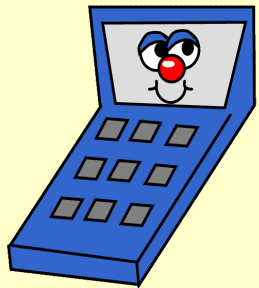# KidZone.ws My Magic Calculator!

Appropriate for Grades 5 and up  (people who are learning to use a calculator)

Impress your friends and give them a little practice using a calculator with this mathematical magic trick.

You could also do this without a calculator for kids learning long multiplication problems, but it's a BIG job.Tell your friend to pick their favorite number between 1 and 9, but don't tell you what it is! Tell them that through the magic of their calculator, you will show them your number. Using the calculator, have them multiply their number by 9. Now have them multiply that number by 12,345,679.    (1 thru 9, skipping the 8) TAH DAH... The calculator shows them their number -- 9 times!

#### Example 1:

Favorite number = 5

5 x 9 = 45

45 x 12345679 = 555,555,555

#### Example 2:

Favorite number = 9

9 x 9 = 81

81 x 12345679 = 999,999,999